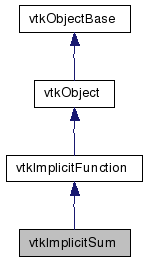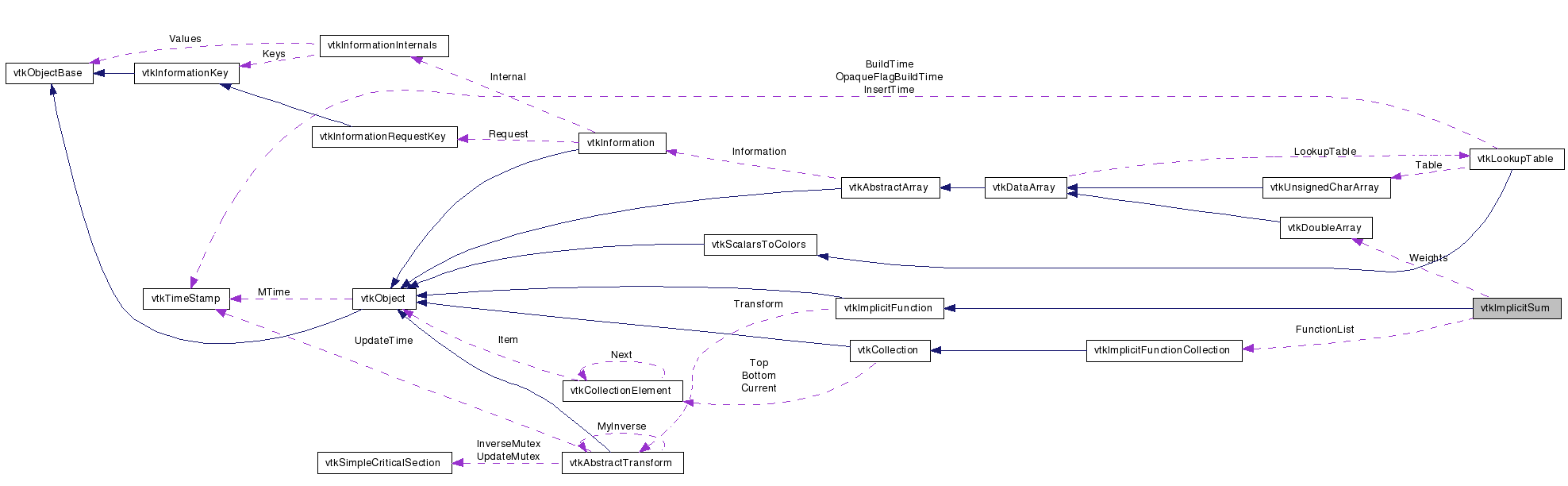vtkImplicitSum Class Reference

#include <vtkImplicitSum.h>

Inheritance diagram for vtkImplicitSum:[legend]
Collaboration diagram for vtkImplicitSum:[legend]

Detailed Description

implicit sum of other implicit functions

vtkImplicitSum produces a linear combination of other implicit functions. The contribution of each function is weighted by a scalar coefficient. The NormalizeByWeight option normalizes the output so that the scalar weights add up to 1. Note that this function gives accurate sums and gradients only if the input functions are linear.

Tests:
vtkImplicitSum (Tests)

Definition at line 36 of file vtkImplicitSum.h.

Public Types

typedef vtkImplicitFunction Superclass

Public Member Functions

virtual const char * GetClassName ()
virtual int IsA (const char *type)
void PrintSelf (ostream &os, vtkIndent indent)
void EvaluateGradient (double x, double g)
unsigned long GetMTime ()
void AddFunction (vtkImplicitFunction *in, double weight)
void RemoveAllFunctions ()
void SetFunctionWeight (vtkImplicitFunction *f, double weight)
double EvaluateFunction (double x)
double EvaluateFunction (double x, double y, double z)
virtual void SetNormalizeByWeight (int)
virtual int GetNormalizeByWeight ()
virtual void NormalizeByWeightOn ()
virtual void NormalizeByWeightOff ()

Static Public Member Functions

static vtkImplicitSumNew ()
static int IsTypeOf (const char *type)
static vtkImplicitSumSafeDownCast (vtkObject *o)

Protected Member Functions

vtkImplicitSum ()
~vtkImplicitSum ()
void CalculateTotalWeight (void)

Protected Attributes

vtkImplicitFunctionCollectionFunctionList
vtkDoubleArrayWeights
double TotalWeight
int NormalizeByWeight

Member Typedef Documentation

Reimplemented from vtkImplicitFunction.

Definition at line 41 of file vtkImplicitSum.h.

Constructor & Destructor Documentation

 vtkImplicitSum::vtkImplicitSum ( ) [protected]

 vtkImplicitSum::~vtkImplicitSum ( ) [protected]

Member Function Documentation

 static vtkImplicitSum* vtkImplicitSum::New ( ) [static]

Create an object with Debug turned off, modified time initialized to zero, and reference counting on.

Reimplemented from vtkObject.

 virtual const char* vtkImplicitSum::GetClassName ( ) [virtual]

Reimplemented from vtkImplicitFunction.

 static int vtkImplicitSum::IsTypeOf ( const char * name ) [static]

Return 1 if this class type is the same type of (or a subclass of) the named class. Returns 0 otherwise. This method works in combination with vtkTypeRevisionMacro found in vtkSetGet.h.

Reimplemented from vtkImplicitFunction.

 virtual int vtkImplicitSum::IsA ( const char * name ) [virtual]

Return 1 if this class is the same type of (or a subclass of) the named class. Returns 0 otherwise. This method works in combination with vtkTypeRevisionMacro found in vtkSetGet.h.

Reimplemented from vtkImplicitFunction.

 static vtkImplicitSum* vtkImplicitSum::SafeDownCast ( vtkObject * o ) [static]

Reimplemented from vtkImplicitFunction.

 void vtkImplicitSum::PrintSelf ( ostream & os, vtkIndent indent ) [virtual]

Methods invoked by print to print information about the object including superclasses. Typically not called by the user (use Print() instead) but used in the hierarchical print process to combine the output of several classes.

Reimplemented from vtkImplicitFunction.

 double vtkImplicitSum::EvaluateFunction ( double x ) [virtual]

Evaluate implicit function using current functions and weights.

Implements vtkImplicitFunction.

 double vtkImplicitSum::EvaluateFunction ( double x, double y, double z ) [inline]

Evaluate implicit function using current functions and weights.

Reimplemented from vtkImplicitFunction.

Definition at line 47 of file vtkImplicitSum.h.

 void vtkImplicitSum::EvaluateGradient ( double x, double g ) [virtual]

Evaluate gradient of the weighted sum of functions. Input functions should be linear.

Implements vtkImplicitFunction.

 unsigned long vtkImplicitSum::GetMTime ( ) [virtual]

Override modified time retrieval because of object dependencies.

Reimplemented from vtkImplicitFunction.

 void vtkImplicitSum::AddFunction ( vtkImplicitFunction * in, double weight )

Add another implicit function to the list of functions, along with a weighting factor.

 void vtkImplicitSum::AddFunction ( vtkImplicitFunction * in ) [inline]

Add another implicit function to the list of functions, weighting it by a factor of 1.

Definition at line 64 of file vtkImplicitSum.h.

 void vtkImplicitSum::RemoveAllFunctions ( )

Remove all functions from the list.

 void vtkImplicitSum::SetFunctionWeight ( vtkImplicitFunction * f, double weight )

Set the weight (coefficient) of the given function to be weight.

 virtual void vtkImplicitSum::SetNormalizeByWeight ( int ) [virtual]

When calculating the function and gradient values of the composite function, setting NormalizeByWeight on will divide the final result by the total weight of the component functions. This process does not otherwise normalize the gradient vector. By default, NormalizeByWeight is off.

 virtual int vtkImplicitSum::GetNormalizeByWeight ( ) [virtual]

When calculating the function and gradient values of the composite function, setting NormalizeByWeight on will divide the final result by the total weight of the component functions. This process does not otherwise normalize the gradient vector. By default, NormalizeByWeight is off.

 virtual void vtkImplicitSum::NormalizeByWeightOn ( ) [virtual]

When calculating the function and gradient values of the composite function, setting NormalizeByWeight on will divide the final result by the total weight of the component functions. This process does not otherwise normalize the gradient vector. By default, NormalizeByWeight is off.

 virtual void vtkImplicitSum::NormalizeByWeightOff ( ) [virtual]

When calculating the function and gradient values of the composite function, setting NormalizeByWeight on will divide the final result by the total weight of the component functions. This process does not otherwise normalize the gradient vector. By default, NormalizeByWeight is off.

 void vtkImplicitSum::CalculateTotalWeight ( void ) [protected]

Member Data Documentation

Definition at line 87 of file vtkImplicitSum.h.

 vtkDoubleArray* vtkImplicitSum::Weights [protected]

Definition at line 88 of file vtkImplicitSum.h.

 double vtkImplicitSum::TotalWeight [protected]

Definition at line 89 of file vtkImplicitSum.h.

 int vtkImplicitSum::NormalizeByWeight [protected]

Definition at line 92 of file vtkImplicitSum.h.

The documentation for this class was generated from the following file:

Generated on Wed Jun 3 19:12:19 2009 for VTK by1.5.6